Скачать презентацию MCS 312 NP Completeness and Approximation algorithms Instructor

292a56a12493c8b41e35417c558dea4a.ppt

• Количество слайдов: 38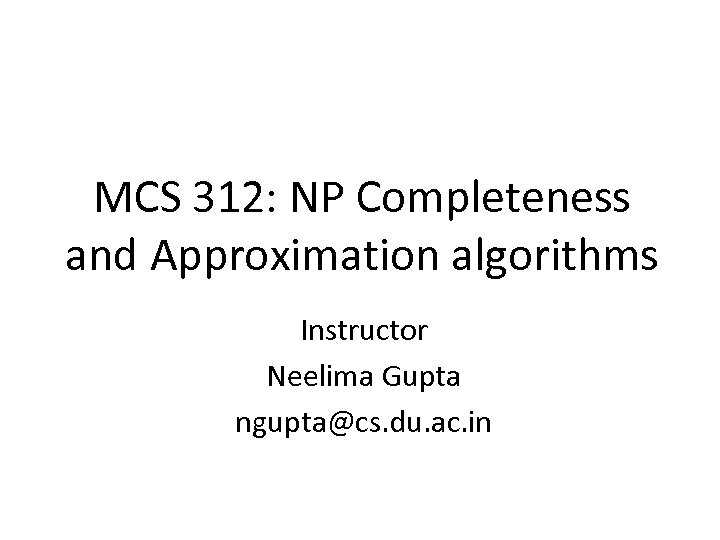MCS 312: NP Completeness and Approximation algorithms Instructor Neelima Gupta [email protected] du. ac. in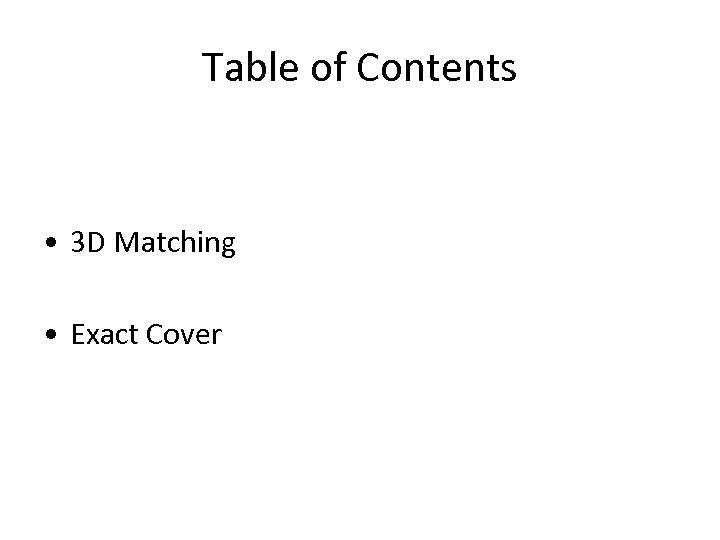Table of Contents • 3 D Matching • Exact Cover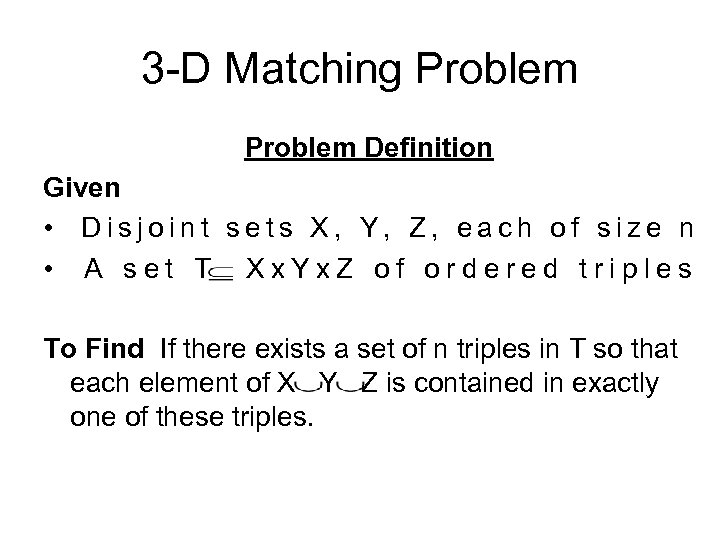3 -D Matching Problem Definition Given • Disjoint sets X, Y, Z, each of size n • A set T Xx. Yx. Z of ordered triples To Find If there exists a set of n triples in T so that each element of X Y Z is contained in exactly one of these triples.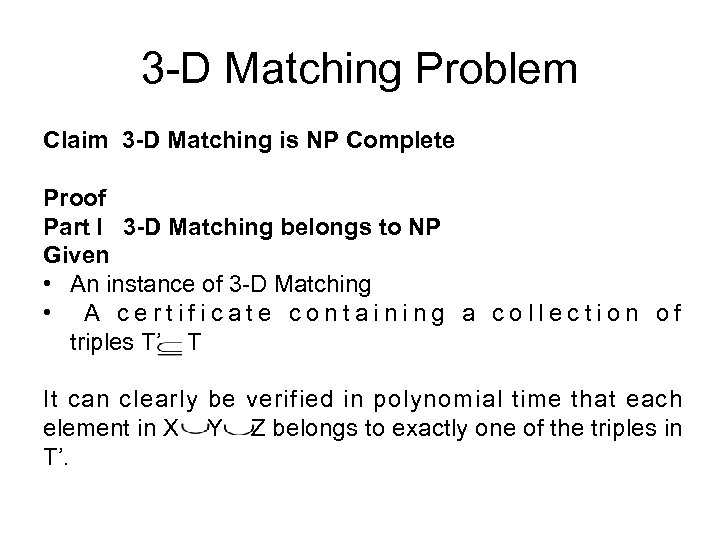3 -D Matching Problem Claim 3 -D Matching is NP Complete Proof Part I 3 -D Matching belongs to NP Given • An instance of 3 -D Matching • A certificate containing a collection of triples T’ T It can clearly be verified in polynomial time that each element in X Y Z belongs to exactly one of the triples in T’.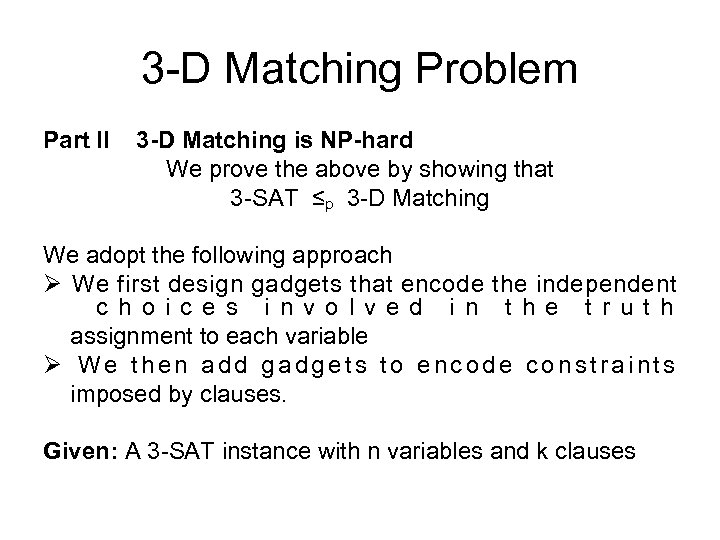3 -D Matching Problem Part II 3 -D Matching is NP-hard We prove the above by showing that 3 -SAT ≤p 3 -D Matching We adopt the following approach Ø We first design gadgets that encode the independent c h o i c e s i n v o l v e d i n t h e t r u t h assignment to each variable Ø We then add gadgets to encode constraints imposed by clauses. Given: A 3 -SAT instance with n variables and k clauses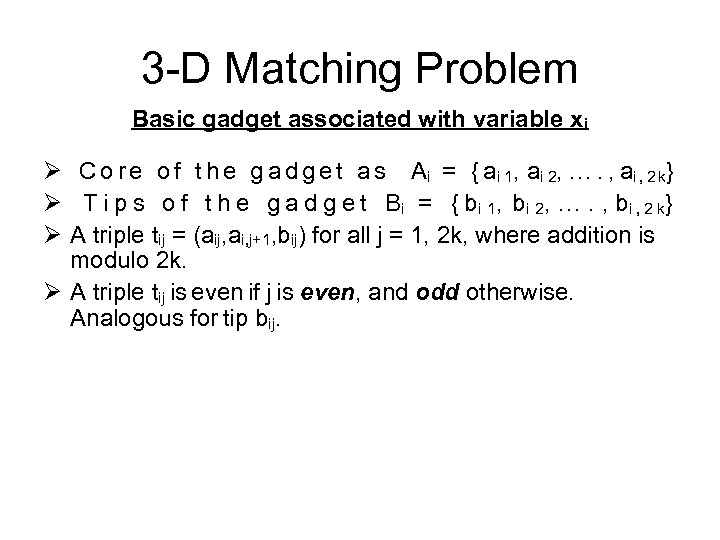3 -D Matching Problem Basic gadget associated with variable xi Ø C o r e o f t h e g a d g e t a s A i = { a i 1 , a i 2 , …. , a i , 2 k} Ø T i p s o f t h e g a d g e t Bi = { bi 1, bi 2, …. , bi , 2 k} Ø A triple tij = (aij, ai, j+1, bij) for all j = 1, 2 k, where addition is modulo 2 k. Ø A triple tij is even if j is even, and odd otherwise. Analogous for tip bij.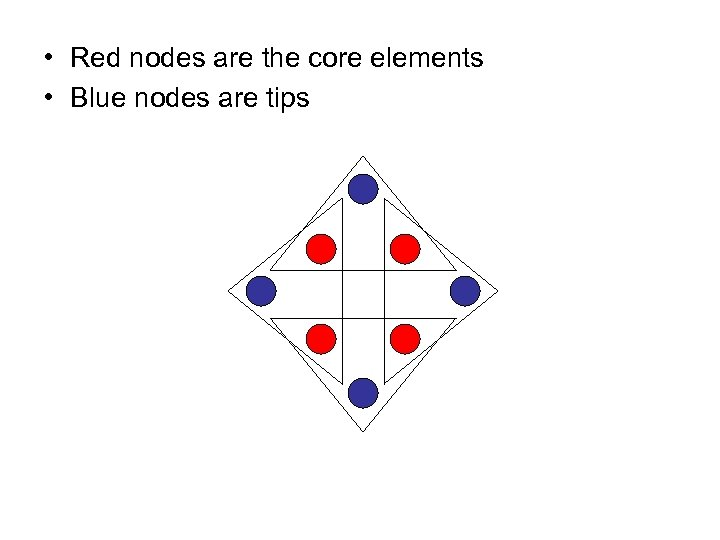• Red nodes are the core elements • Blue nodes are tips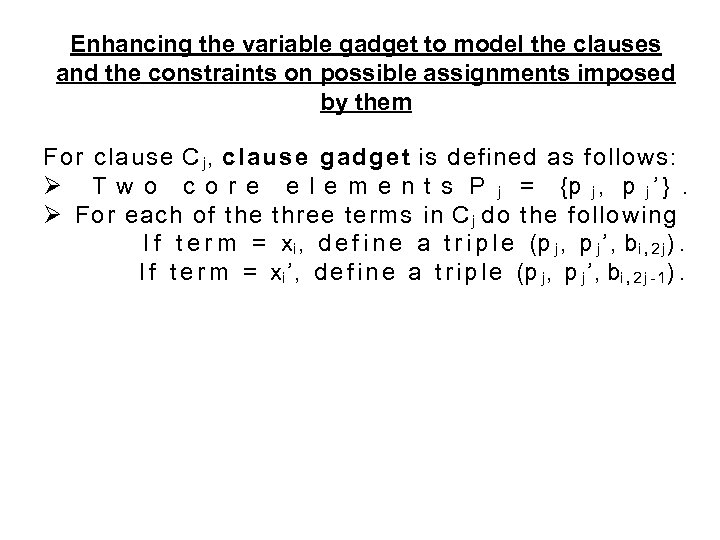Enhancing the variable gadget to model the clauses and the constraints on possible assignments imposed by them For clause C j, clause gadget is defined as follows: Ø T w o c o r e e l e m e n t s P j = {p j , p j ’ }. Ø For each of the three terms in C j do the following I f t e r m = xi , d e f i n e a t r i p l e (p j , p j ’, bi , 2 j). I f t e r m = xi ’, d e f i n e a t r i p l e (p j , p j ’, bi , 2 j - 1).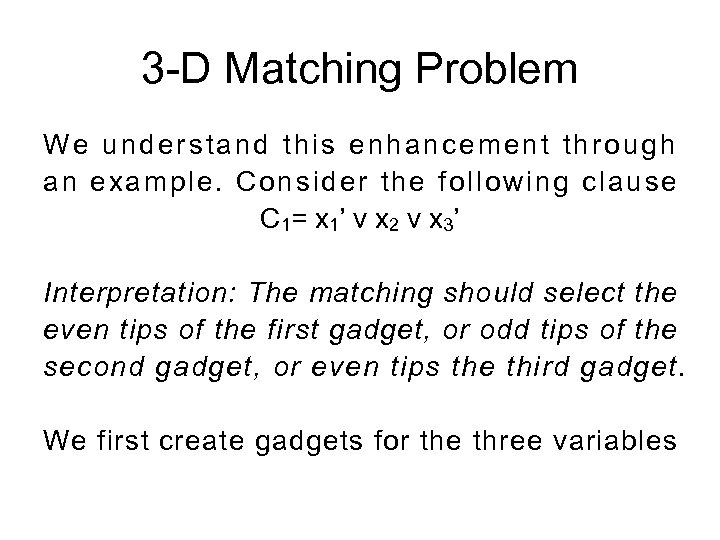3 -D Matching Problem We understand this enhancement through an example. Consider the following clause C 1= x 1’ v x 2 v x 3’ Interpretation: The matching should select the even tips of the first gadget, or odd tips of the second gadget, or even tips the third gadget. We first create gadgets for the three variables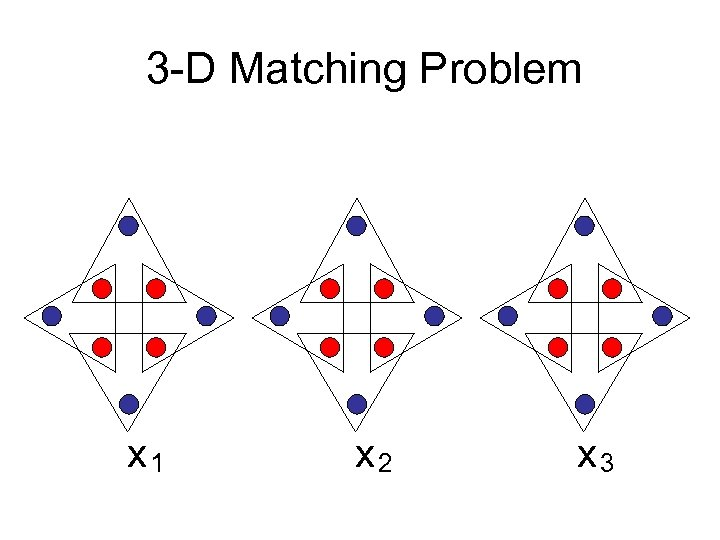3 -D Matching Problem x 1 x 2 x 3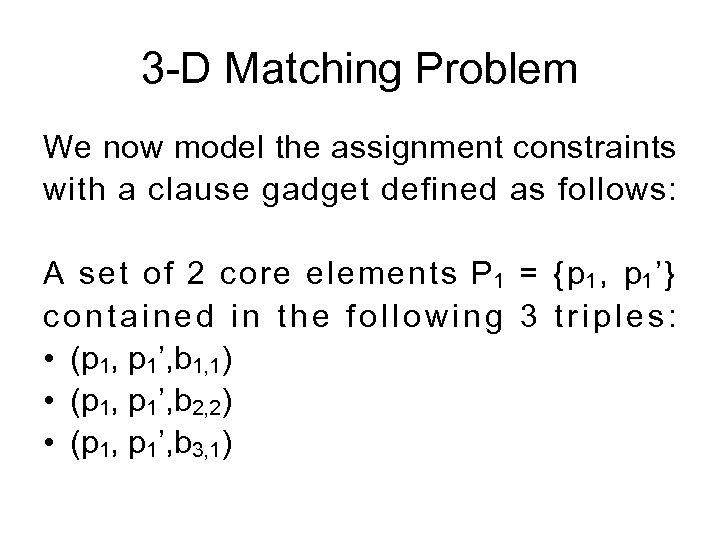3 -D Matching Problem We now model the assignment constraints with a clause gadget defined as follows: A set of 2 core elements P 1 = { p 1 , p 1 ’} contained in the following 3 triples: • (p 1, p 1’, b 1, 1) • (p 1, p 1’, b 2, 2) • (p 1, p 1’, b 3, 1)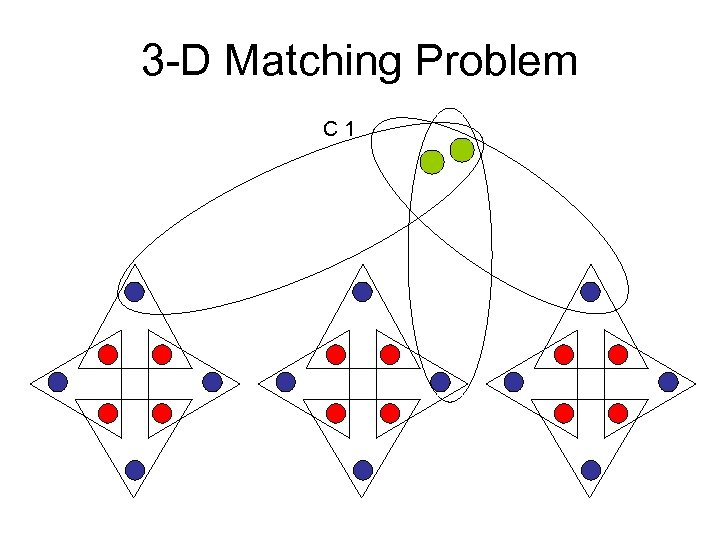3 -D Matching Problem C 1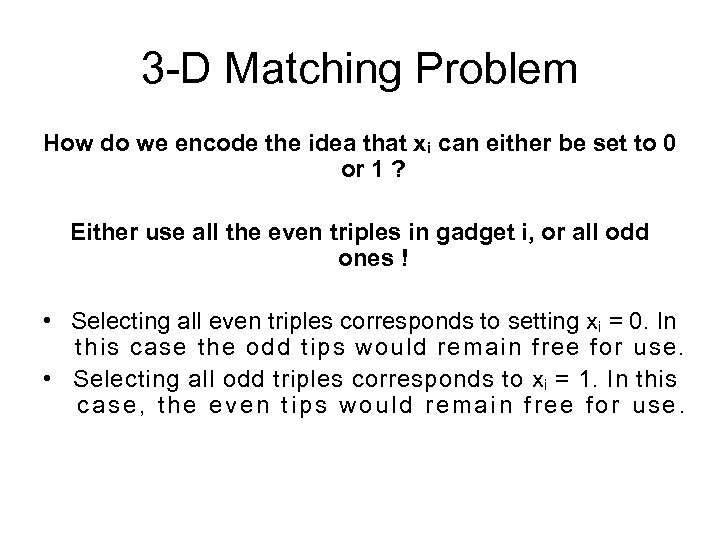3 -D Matching Problem How do we encode the idea that xi can either be set to 0 or 1 ? Either use all the even triples in gadget i, or all odd ones ! • Selecting all even triples corresponds to setting xi = 0. In this case the odd tips would remain free for use. • Selecting all odd triples corresponds to xi = 1. In this case, the even tips would remain free for use.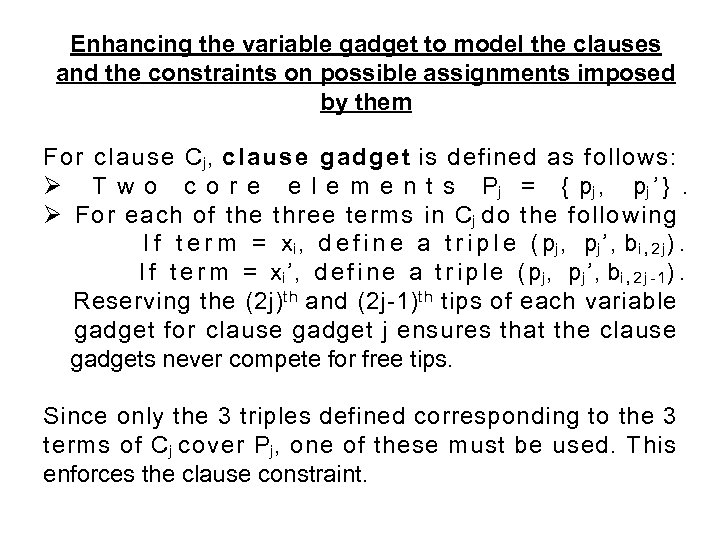Enhancing the variable gadget to model the clauses and the constraints on possible assignments imposed by them For clause Cj, clause gadget is defined as follows: Ø T w o c o r e e l e m e n t s P j = { pj , pj ’ }. Ø For each of the three terms in Cj do the following I f t e r m = xi , d e f i n e a t r i p l e ( pj , pj ’, bi , 2 j). I f t e r m = xi ’, d e f i n e a t r i p l e ( pj , pj ’, bi , 2 j - 1). Reserving the (2 j)th and (2 j-1)th tips of each variable gadget for clause gadget j ensures that the clause gadgets never compete for free tips. Since only the 3 triples defined corresponding to the 3 terms of Cj cover Pj, one of these must be used. This enforces the clause constraint.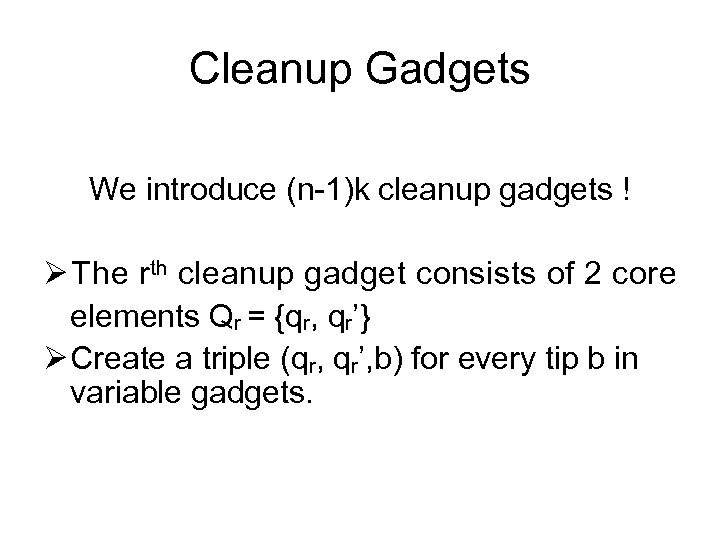Cleanup Gadgets We introduce (n-1)k cleanup gadgets ! Ø The rth cleanup gadget consists of 2 core elements Qr = {qr, qr’} Ø Create a triple (qr, qr’, b) for every tip b in variable gadgets.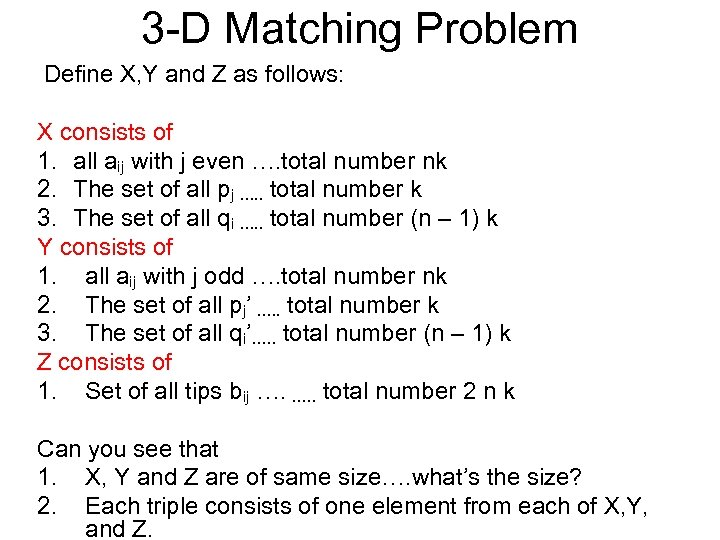3 -D Matching Problem Define X, Y and Z as follows: X consists of 1. all aij with j even …. total number nk 2. The set of all pj …. . total number k 3. The set of all qi …. . total number (n – 1) k Y consists of 1. all aij with j odd …. total number nk 2. The set of all pj’ …. . total number k 3. The set of all qi’…. . total number (n – 1) k Z consists of 1. Set of all tips bij …. …. . total number 2 n k Can you see that 1. X, Y and Z are of same size…. what’s the size? 2. Each triple consists of one element from each of X, Y, and Z.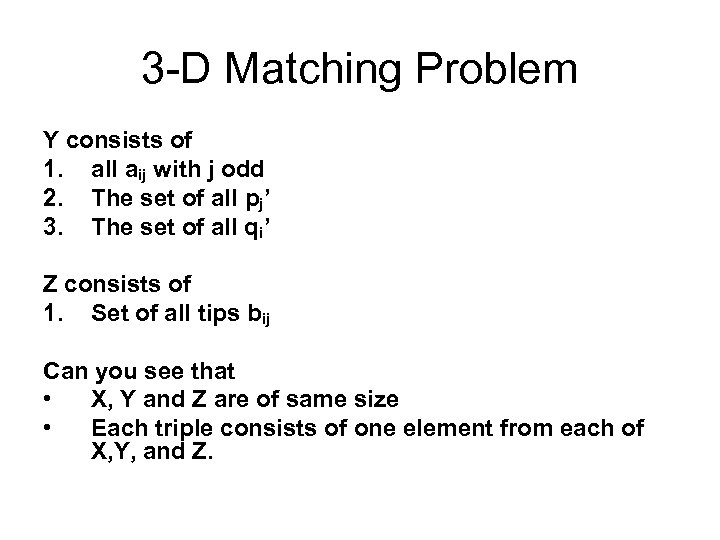3 -D Matching Problem Y consists of 1. all aij with j odd 2. The set of all pj’ 3. The set of all qi’ Z consists of 1. Set of all tips bij Can you see that • X, Y and Z are of same size • Each triple consists of one element from each of X, Y, and Z.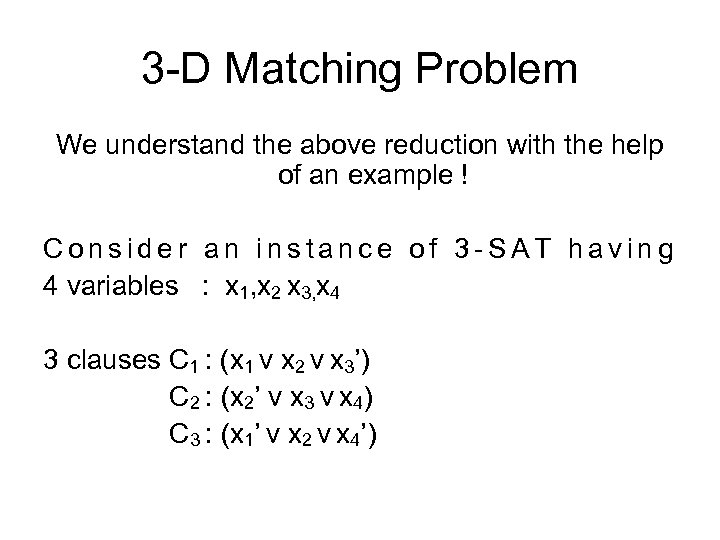3 -D Matching Problem We understand the above reduction with the help of an example ! Consider an instance of 3 -SAT having 4 variables : x 1, x 2 x 3, x 4 3 clauses C 1 : (x 1 v x 2 v x 3’) C 2 : (x 2’ v x 3 v x 4) C 3 : (x 1’ v x 2 v x 4’)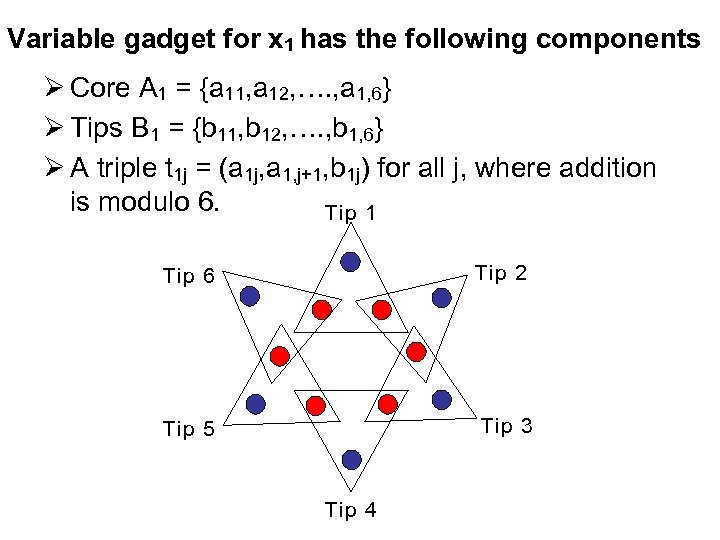Variable gadget for x 1 has the following components Ø Core A 1 = {a 11, a 12, …. , a 1, 6} Ø Tips B 1 = {b 11, b 12, …. , b 1, 6} Ø A triple t 1 j = (a 1 j, a 1, j+1, b 1 j) for all j, where addition is modulo 6. Tip 1 Tip 6 Tip 2 Tip 5 Tip 3 Tip 4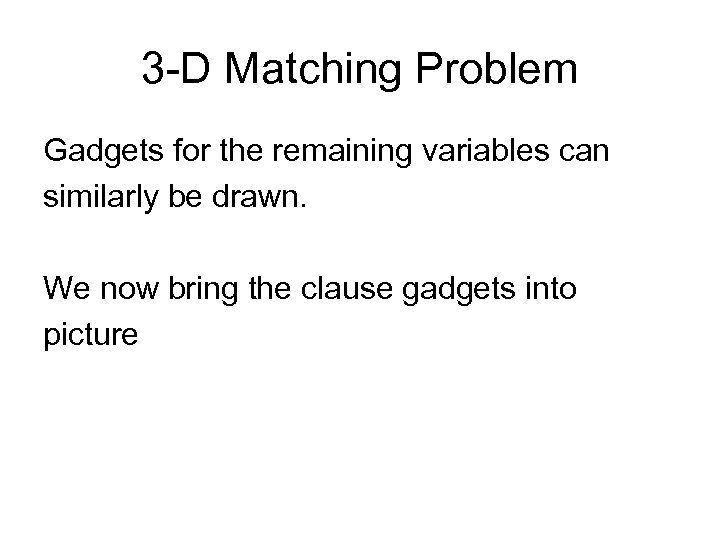3 -D Matching Problem Gadgets for the remaining variables can similarly be drawn. We now bring the clause gadgets into picture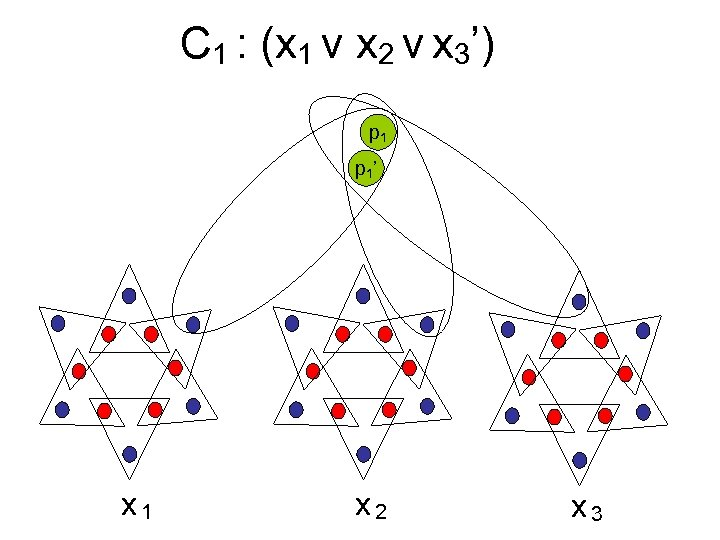C 1 : (x 1 v x 2 v x 3’) p 1 ’ x 1 x 2 x 3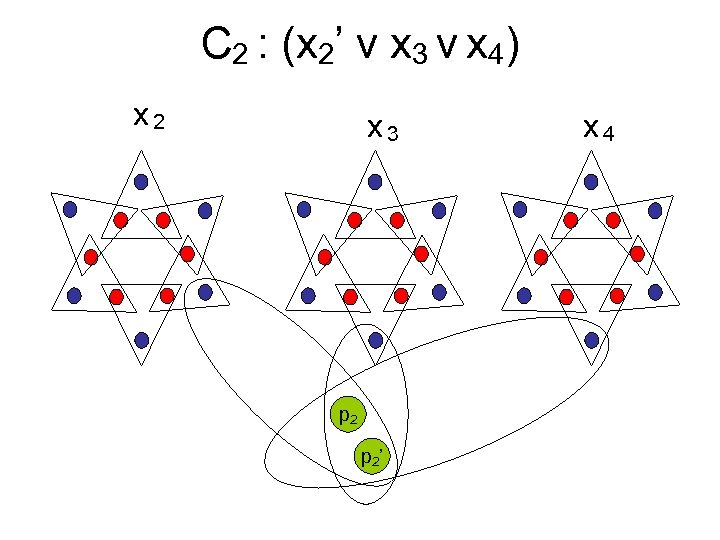C 2 : (x 2’ v x 3 v x 4) x 2 x 3 p 2 ’ x 4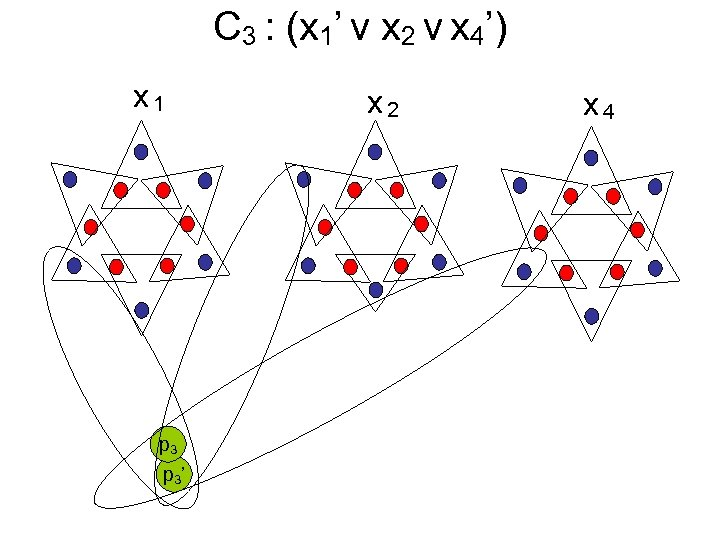C 3 : (x 1’ v x 2 v x 4’) x 1 p 3 ’ x 2 x 4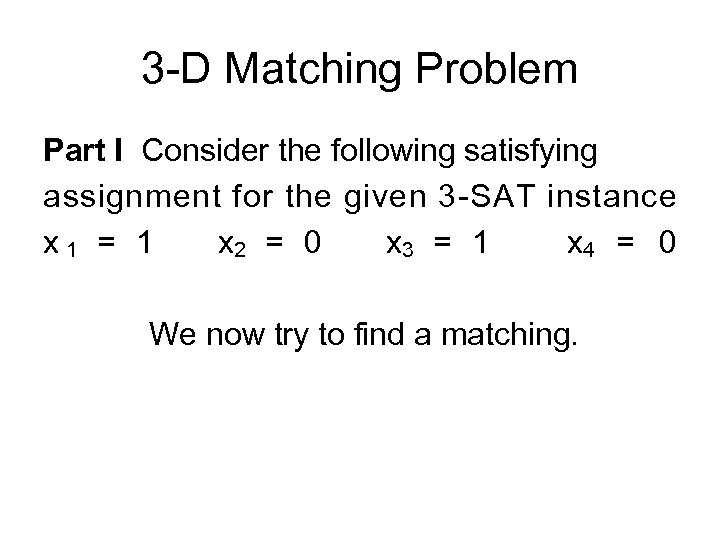3 -D Matching Problem Part I Consider the following satisfying assignment for the given 3 -SAT instance x 1 = 1 x 2 = 0 x 3 = 1 x 4 = 0 We now try to find a matching.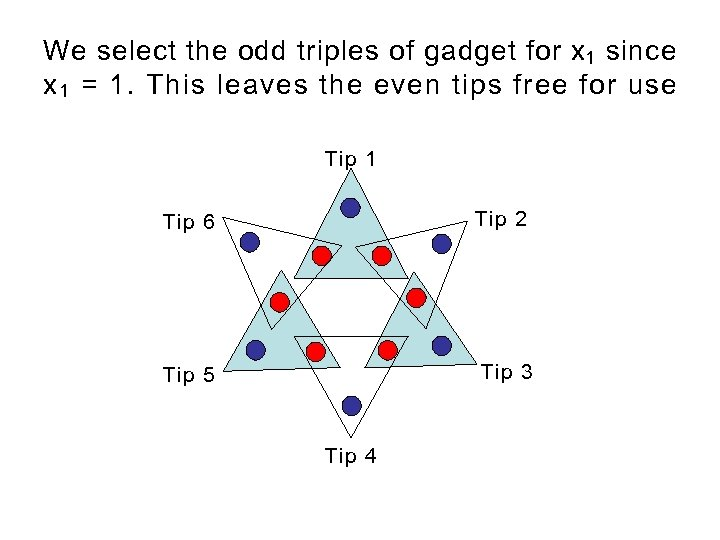We select the odd triples of gadget for x 1 since x 1 = 1. This leaves the even tips free for use Tip 1 Tip 6 Tip 2 Tip 5 Tip 3 Tip 4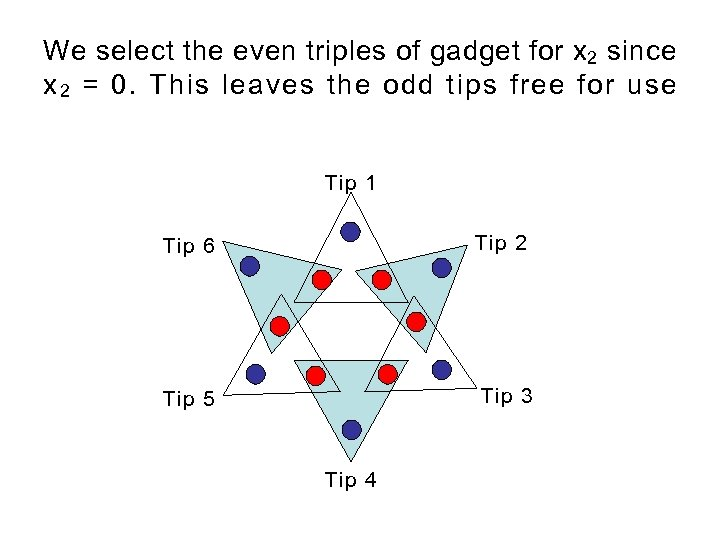We select the even triples of gadget for x 2 since x 2 = 0. This leaves the odd tips free for use Tip 1 Tip 6 Tip 2 Tip 5 Tip 3 Tip 4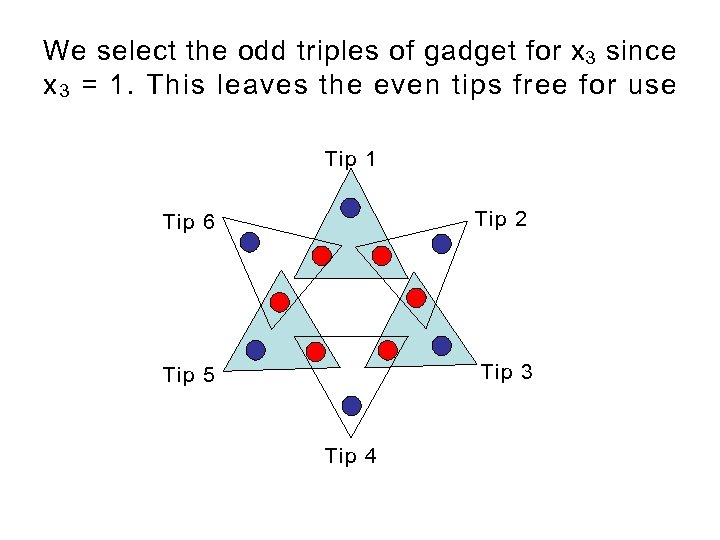We select the odd triples of gadget for x 3 since x 3 = 1. This leaves the even tips free for use Tip 1 Tip 6 Tip 2 Tip 5 Tip 3 Tip 4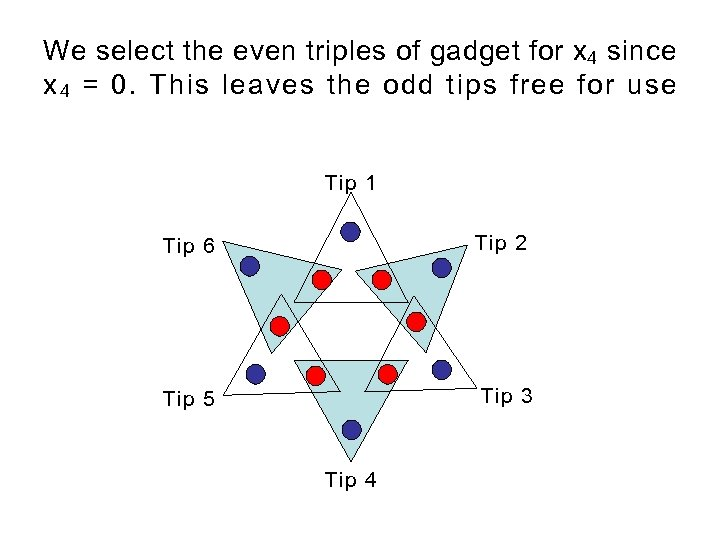We select the even triples of gadget for x 4 since x 4 = 0. This leaves the odd tips free for use Tip 1 Tip 6 Tip 2 Tip 5 Tip 3 Tip 4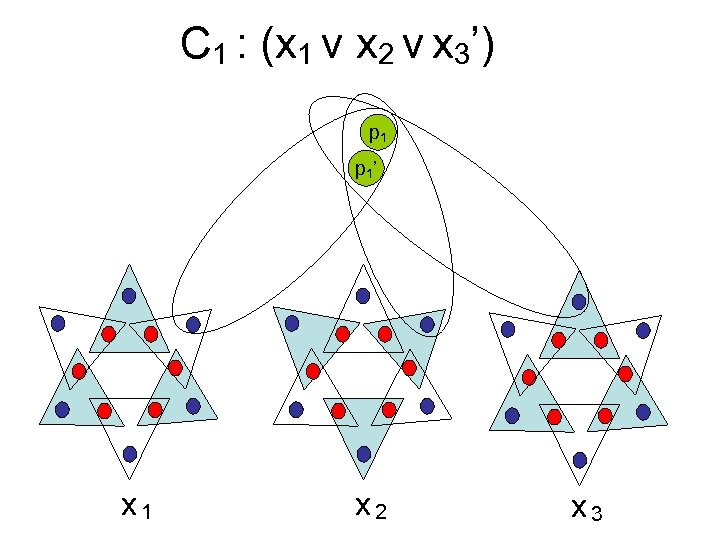C 1 : (x 1 v x 2 v x 3’) p 1 ’ x 1 x 2 x 3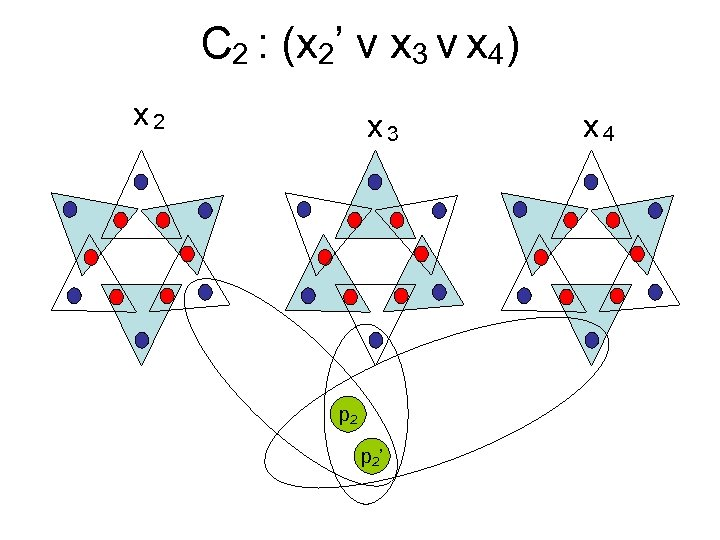C 2 : (x 2’ v x 3 v x 4) x 2 x 3 p 2 ’ x 4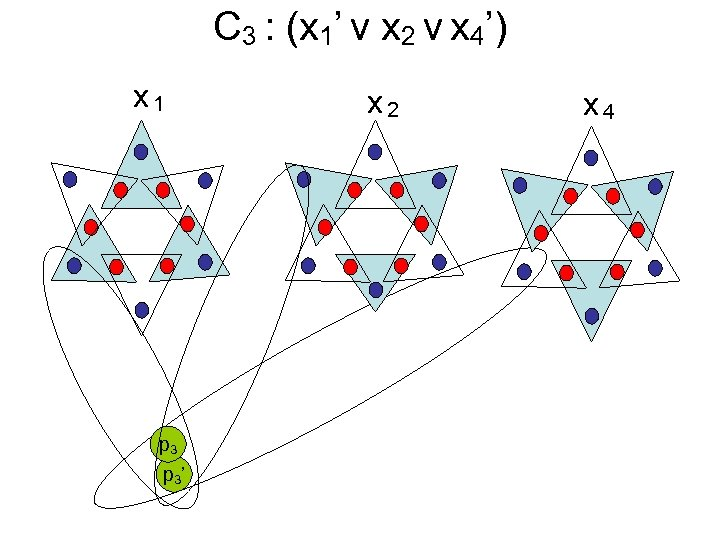C 3 : (x 1’ v x 2 v x 4’) x 1 p 3 ’ x 2 x 4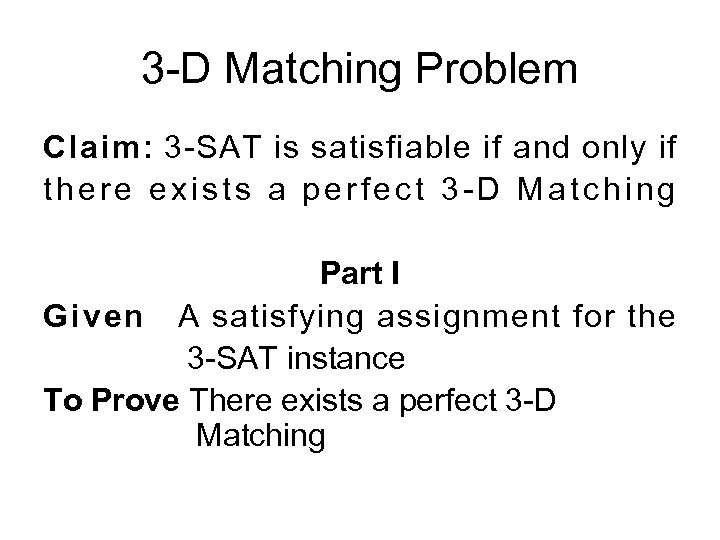3 -D Matching Problem Claim: 3 -SAT is satisfiable if and only if there exists a perfect 3 -D Matching Part I Given A satisfying assignment for the 3 -SAT instance To Prove There exists a perfect 3 -D Matching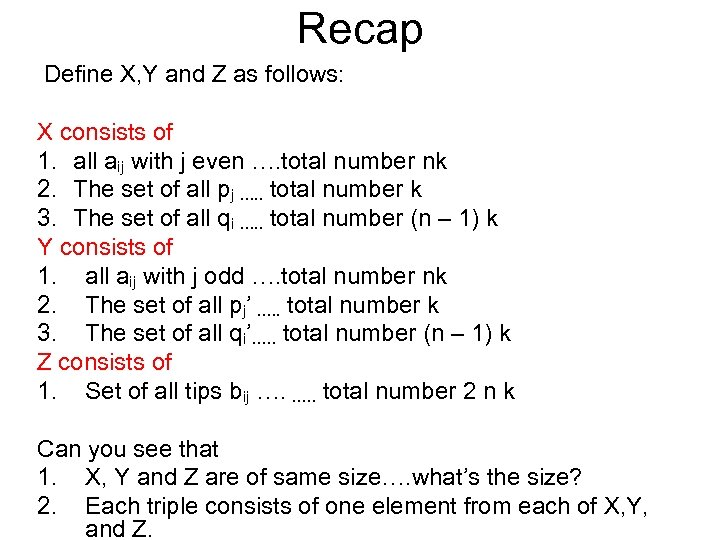Recap Define X, Y and Z as follows: X consists of 1. all aij with j even …. total number nk 2. The set of all pj …. . total number k 3. The set of all qi …. . total number (n – 1) k Y consists of 1. all aij with j odd …. total number nk 2. The set of all pj’ …. . total number k 3. The set of all qi’…. . total number (n – 1) k Z consists of 1. Set of all tips bij …. …. . total number 2 n k Can you see that 1. X, Y and Z are of same size…. what’s the size? 2. Each triple consists of one element from each of X, Y, and Z.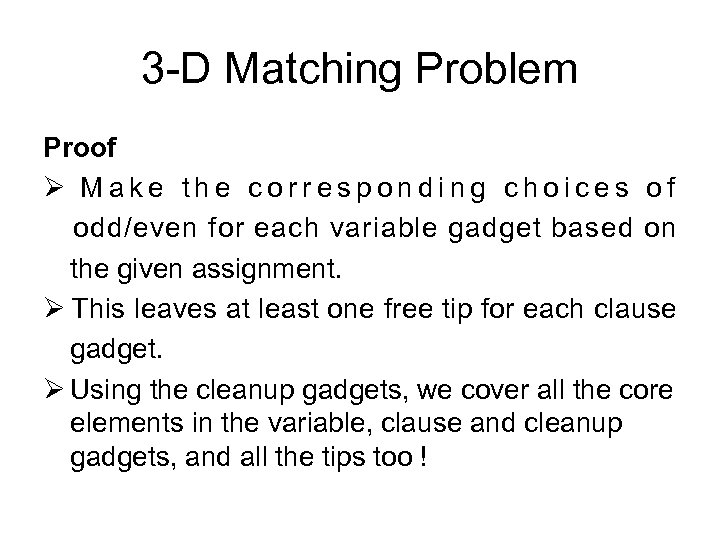3 -D Matching Problem Proof Ø Make the corresponding choices of odd/even for each variable gadget based on the given assignment. Ø This leaves at least one free tip for each clause gadget. Ø Using the cleanup gadgets, we cover all the core elements in the variable, clause and cleanup gadgets, and all the tips too !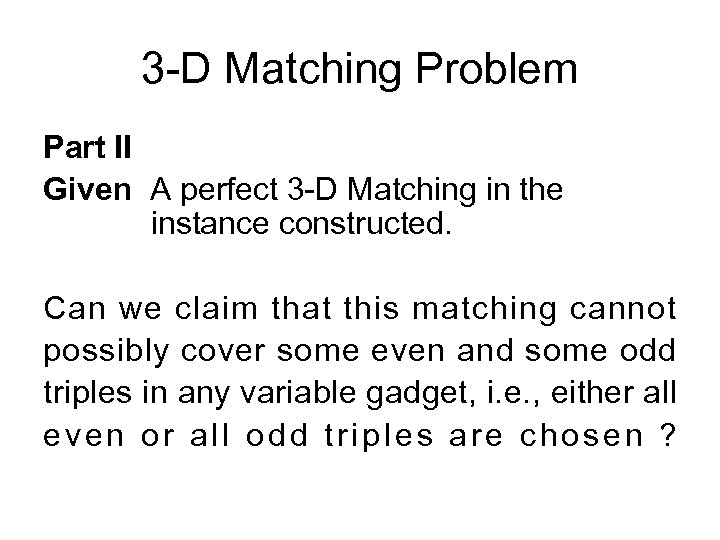3 -D Matching Problem Part II Given A perfect 3 -D Matching in the instance constructed. Can we claim that this matching cannot possibly cover some even and some odd triples in any variable gadget, i. e. , either all even or all odd triples are chosen ?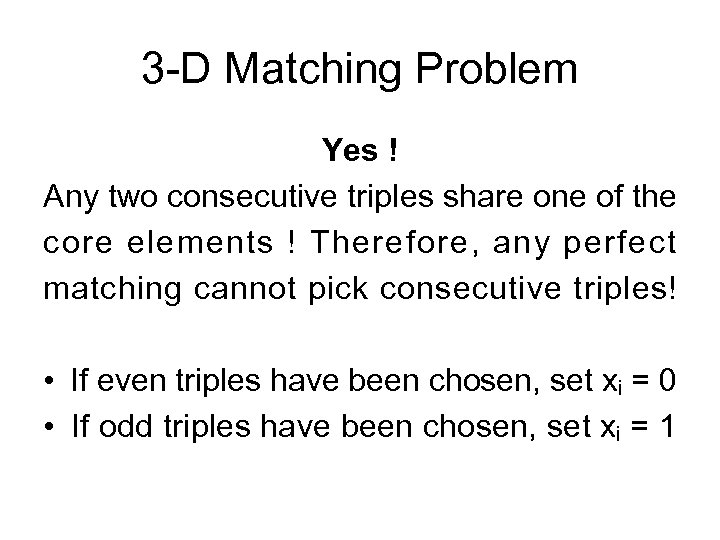3 -D Matching Problem Yes ! Any two consecutive triples share one of the core elements ! Therefore, any perfect matching cannot pick consecutive triples! • If even triples have been chosen, set xi = 0 • If odd triples have been chosen, set xi = 1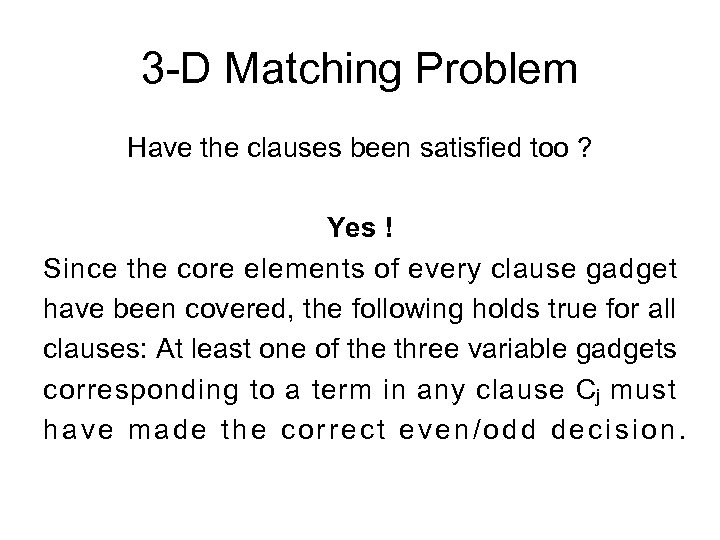3 -D Matching Problem Have the clauses been satisfied too ? Yes ! Since the core elements of every clause gadget have been covered, the following holds true for all clauses: At least one of the three variable gadgets corresponding to a term in any clause Cj must have made the correct even/odd decision.Big Thanks to Sonika Arora